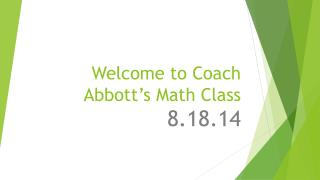Download PresentationWelcome to Coach Abbott’s Math Class

# Welcome to Coach Abbott’s Math Class - PowerPoint PPT PresentationDownload Presentation## Welcome to Coach Abbott’s Math Class

- - - - - - - - - - - - - - - - - - - - - - - - - - - E N D - - - - - - - - - - - - - - - - - - - - - - - - - - -
##### Presentation Transcript

1. Focus • -72 + (-54) = • -3.5 – (-7.9) = • 1/2 -3/4 = • 62 –(+23) + (-27) –(-18) = • Give an example for the following properties…. • Associative of addition, commutative of multiplication, distributive property of multiplication

2. Focus ext. • -7.4 + (-2.4) = • -49 – (-.37) = • -42 - 34 = • 32 –(-46) + (-29) –(-44) = • Why are

3. Standards • The Number System 6.NS • Apply and extend previous understandings of multiplication and division to divide fractions by fractions. • MCC6.NS.1 Interpret and compute quotients of fractions, and solve word problems involving division of • fractions by fractions, Compute fluently with multi‐digit numbers and find common factors and multiples. • MCC6.NS.2 Fluently divide multi‐digit numbers using the standard algorithm. • MCC6.NS.3 Fluently add, subtract, multiply, and divide multi‐digit decimals using the standard algorithm for • each operation. • MCC6.NS.4 Find the greatest common factor of two whole numbers less than or equal to 100 and the least • common multiple of two whole numbers less than or equal to 12. Use the distributive property to express a sum • of two whole numbers 1–100 with a common factor as a multiple of a sum of two whole numbers with no • common factor. For example, express 36 + 8 as 4 (9 + 2).

4. Agenda • Focus • Folders / #1 counter worksheet • Target- • Practice – COACH where you • Enrichment - • Problem Solving / Coach where you are

5. Tier Time Options • Work on Edgenuity on your own device • Math work • Ask me or another teacher for help on an issue • Brainstorm your BIG IDEA for a PROJECT • Read quietly

6. Yesterday's Focus Question

7. So far and looking ahead • After this week on Aug 18th • Students have had… • 10 days of school • 12-15 hours of lab time • If you are still completing 6th grade Edgenuity content, how far are you? • Each student that had finished 7th grade has started with 17 lessons, how far are you? • 4 weeks, 20 school days left in this unit. • You will have class 8 times plus 4 flex fridays One lesson a day keeps you on track.

8. Edgenuity • I will check progress Friday • Monitor your own progress: completion date is 9.15 • Must complete 6th grade work • Edgenuity progress will effect assignments in class • Pretest Passing > 90% Quiz > 80% • Retakes: I have three retakes for you on a quiz • Sometime that includes the pretest, sometimes it doesn’t • Assume you have 2-3 quiz attempts • When you run out of attempts I will send you back through the assignment • Email my regular email

9. Next 2 weeks • PROGRESS TRACKING SHEET • Operations with Rational Numbers unit • Week 3 • Number line • absolute value • rational #s • adding subtracting multiplying dividing of integers • Week 4 • Operations with Integers • Problem Solving with Integers

10. Last 2 weeks of unit • Week 5 • Rational #s • adding/subtracting decimals • multiplying/dividing decimals • problem solving decimals • Week 6 • adding/subtracting fractions • multiplying/dividing fractions • problem solving fractions • Friday Unit Test and Projects 9.12 • You have until Monday 9.15 to complete on target date

11. TO DO • Problem Solving and Operations with Integers • Order of Operations • PEMDAS • Problem Solving and Operations with Decimals • Problem Solving and Operations with Fractions

12. Focus Question • What questions do you have for me??

13. Folder Review • Copy NOTES • Complete PRACTICE • Show Coach Abbott completion and I will give you 1 more attempts • If you fail this quiz you will be referred to Ms. Grayer

14. Coach book Preview or Review: • Copy UNDERSTANDING • Copy and understand CONNECT • Complete TRY • Copy and understand an EXAMPLE • Complete PROBLEM SOLVING • Complete PRACTICE questions (odd only) • Show Coach Abbott work and progress sheet, get book and check answers

15. Enrichment • Problem Solvers • #3 Tasks • Continue to move ahead on Edgenuity • Practice old skills • Peer tutor another student